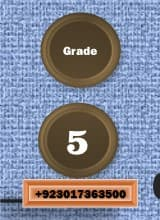0
304We believe that the best way to learn Mathematics is to practice as many exercises as possible Dear Students, here several Printable worksheets have been created, separated by themes, so that each student can practice in the subjects where he / she feels the most difficulty. Please, Free Download Math Addition Printable worksheets, multiple digit addition worksheets, no-carrying addition worksheets and other addition topics. These addition worksheets are free for you or class work use.

Subtraction Worksheets

Here you can free download Printable subtraction worksheets. Many topics of Spaceship Math Subtraction worksheets, timed subtraction tests, multiple digit subtraction worksheets, simple borrowing and regrouping worksheets, and math worksheets with mixed addition and subtraction problems

Multiplication Worksheets

Dear Students, Multiplication Printable Grade 5 worksheets. Put your fingers away, because this is the first math operation where memorization of the facts is a requirement. You’ll find multiplication worksheets for Simple Rules for Mastering the Times Table, multiple digit multiplication, squares and other multiplication worksheet topics. All of these multiplication worksheets Comprise answer keys and are at once  printable and ready classroom or home school use.

Division Worksheets

Dear Students, Grade 5 Division worksheets can download here free of cost . This includes Shuttle Math Division worksheets, multiple digit division worksheets, square root worksheets, cube roots, mixed multiplication, free division worksheets, Beginning division worksheet and division worksheets. Dear You can free download Grade 5 worksheets.

Pr-Algebra Word Problems

Pr-algebra addition, subtraction, Algebraic Geometry,  Algebraic multiplication and division word problems dealing with relationships between numbers in simple equations… A great first step for easing into algebra!

Percentages

Many Math Printable Worksheets to practice using and calculating percentages of other numbers, including converting between fractions and percentages.

Number Patterns

These number patterns worksheets help students develop the needed skills for determining patterns and relationships between numbers.